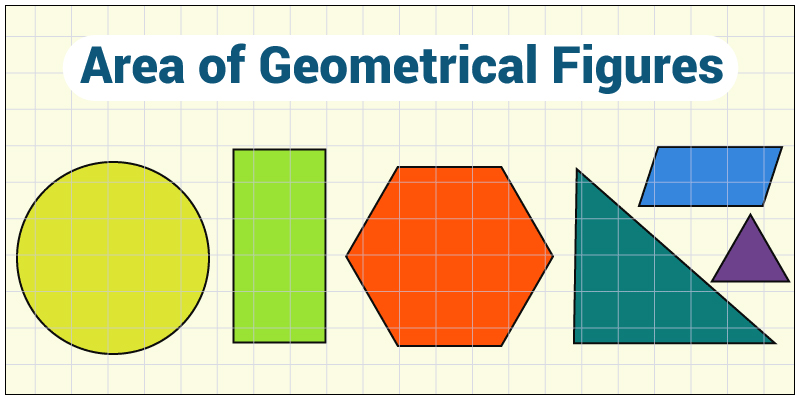# GMAT Quant: Geometry – AreaArea is the space that is confined within any shape. There are many standard figures who has a fixed formula for calculating their areas, while some needs to be broken down to two or more shape so that their area can be calculated.

Let’s check some standard formulas for some of the shapes:

Area of a Triangle

$Area = \frac{1}{2} \times b \times h$

Area of a Circle

$Area = \pi r^{2}$

Area of a Square

$Area = (side)^{2}$

Area of a Rectangle

$Area = Length \times Width$

Area of a Parallelogram

$Area = Base \times Height$

Also Read: GMAT Quant: Arithmetic – Numbers

Area of Trapezoid

$Area = 5 \times (base_1 + base_2) \times height$

Area of a Rhombus

Rhombus is a shape whose all sides are equal but only opposite sides are parallel to one another. The diagonals of rhombus bisect at 90°.

$Area = 5 \times diagonal_1 \times diagonal_2$

Let’s solve a question and see how easy calculating area is:

Question: A square of area 81 sq. units, made of a thin wire, is converted into semi-circle. What is the area of the semi-circle?

1. 49
2. 77
3. 154
4. 84
5. 98

Solution:

Perimeter of square = Perimeter of semi-circle     (Equation 1)

$Area \; of \; square = a^2 = 81$

So, a = 9

Perimeter of square = 9 × 4 = 36

From Equation 1,

$\pi r + 2 r = 36$ $\frac{22}{7} r + 2 r = 36$ $\frac{36 r}{7} = 36$

So, $r = 7$

Area of semi-circle =  $A = \frac{\pi r^2}{2}$

Area = $A = \frac{22}{7} \times 7 \times 7 \times \frac{1}{2} = 77 \; sq. \; units$

Read more GMAT Quant related articles.

We’ll be glad to help you in your GMAT preparation journey. You can ask for any assistance related to GMAT and MBA from us by calling us at +918884544444. You can write to us at gmat@byjus.com.